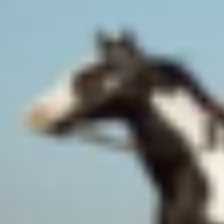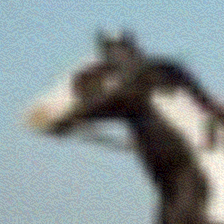# UIUCTF 2021 - pwnies please

##### by sera

Disguise these pwnies to get the flag!

note: first solve gets \$100 from ian (unintended solves don’t count)

author: Anusha Ghosh, Akshunna Vaishnav, ian5v, Vanilla

## Introduction

This challenge is a AI related challenge where we need to perform an adversial attack against an image classification AI model in order to fool it without changing the image “too much”.

I know almost nothing about AI, but I was able to solve this challenge anyway utilizing some good resources online, and I’ll walk through how I did it without specific AI knowledge.

## The challenge

When visiting the page, we are given a challenge image of a horse and asked to disguise it and upload it again. In particular, we need to fool a provided model (the bouncer) but not fool the “robust” model, which is not provided. We also cannot change the image too much according to a measurement based on the image’s data.

When we pass an image, a counter stored in the session is incremented, and a fail counter is reset. If we fail over 3 images in a row, our progress is reset, but if we pass 50 then we get the flag!

## The neural network

The provided website source includes all the code needed to utilize the model. The `get_prediction` function feeds an image into the classifier, so we can use that as an example of how to use the model.

We can see that it performs these steps:

• Perform a normalization in the image (transform_image)
• Runs the model on the image (`outputs = model(image)`)
• Grabs the classification from the model
• Returns the classification if the image didn’t change that much from the original…

Seeing the code, we’ll need to install the neural network library used:

``````python3 -m pip install pytorch
``````

## The attack

Googling “pytorch adversial attack”, we can see find a python module called torchattacks that can implement the attack for us. One of the examples fits our use case personally - we want to fool a resnet18 classifier (whatever resnet18 even means).

``````python3 -m pip install torchattacks
``````

Although the code won’t work as is, I followed the steps in this example to create a solution. (You may want open it up in another tab to follow along.)

The first thing I did was copy the initialization code from the challenge source:

``````from torchvision import models
import torchvision.transforms as transforms
import torchvision
import torch.nn as nn
import torch
import numpy as np
from PIL import Image
import time
import io

# ------------------ Model goes here ⬇------------------ #
imagenet_class_index = ['plane', 'car', 'bird', 'cat', 'deer', 'dog', 'frog', 'horse', 'ship', 'truck']
device = torch.device("cuda:0" if torch.cuda.is_available() else "cpu")

model_ = models.resnet18()
num_ftrs = model_.fc.in_features
model_.fc = nn.Linear(num_ftrs, len(imagenet_class_index))
model_ft = model_.to(device)

# model.eval() (removed for later)
``````

The example seems to load some kind of folder containing image examples and classifications. We don’t have that, but we have single samples (what the server tells us to modify) and its real classification (horse).

The provided challenge source contains an `image_to_tensor`, so by copying that we can load our png into a tensor - the input to the model.

``````def image_to_tensor(img):
tensor = np.array(img).astype(np.float32) / 255.0
# HWC -> CHW
tensor = tensor.transpose(2, 0, 1)
tensor = tensor[None, :, :, :]  # add batch dimension

images = image_to_tensor(Image.open(open("pwny.png")))
``````

The label is just ‘horse’.

I don’t know what Inception v3 is, but this step seems to composite a normalization layer onto the model. It also uses a custom `Normalization` class claiming `we can't use torch.transforms because it supports only non-batch images.`. However, we don’t seem to need batch images, so I applied the normalization like in the challenge source.

``````norm_layer = transforms.Normalize(mean=[0.485, 0.456, 0.406], std=[0.229, 0.224, 0.225])
model = nn.Sequential(
norm_layer,
model_
).to(device)
model.eval()
``````

The example code for this section looks like it creates a bunch of attacks and executes them all. We’ll only use the first one since we’re lazy.

An attack is instantiated by passing it the model and a paramter `epsilon` which according to Google determines how agressive the modifications are. We can adjust this as needed if the images fail the image difference check.

To use the attack, we just call it on our set of inputs (just one) and original labels (also just one). It took a bit of trial and error to get the label in as wanted – we need to convert the label index to a tensor and feed that in.

The output of the attack seems to be the image, so we can feed that back into the model to see if it fooled the model.

``````labels = torch.tensor([imagenet_class_index.index("horse")])
atk = FGSM(model, eps=8/255)
labels = labels.to(device)
_, pre = torch.max(outputs.data, 1)
print(imagenet_class_index[pre])
with open("pwny_modified.png", "wb") as f:
``````

After running this code, we can see that we generate an image that (may) fool the model. Here is what I get by running the code on an image from the server:

Original Image New Imagehorse plane

The modified image has some visible noise added, and the model thinks it is a ‘plane’. If we upload it to the server, we can see our attack just works!

## Automation

Now that we have a working attack, we can automate the retrieving and submitting of images to the server. To prevent the situation where we modify the image too much, we can iterate the `epsilon` attack paramter until it is just enough to fool the original model.

`requests``Session` module will handle the session cookie for us, we just need to parse the base64 out of the HTML. To avoid saving temporary files, we can use the `BytesIO` module.

## Code

``````from torchvision import models
import torchvision.transforms as transforms
import torchvision
import torch.nn as nn
import torch
import numpy as np
from PIL import Image
import time
import io

# ------------------ Model goes here ⬇------------------ #
imagenet_class_index = ['plane', 'car', 'bird', 'cat', 'deer', 'dog', 'frog', 'horse', 'ship', 'truck']
device = torch.device("cuda:0" if torch.cuda.is_available() else "cpu")

model_ = models.resnet18()
num_ftrs = model_.fc.in_features
model_.fc = nn.Linear(num_ftrs, len(imagenet_class_index))
model_ft = model_.to(device)

norm_layer = transforms.Normalize(mean=[0.485, 0.456, 0.406], std=[0.229, 0.224, 0.225])
model = nn.Sequential(
norm_layer,
model_
).to(device)
model.eval()

def tensor_to_image(tensor):
tensor = tensor.detach().squeeze().numpy()
tensor = tensor.transpose(1, 2, 0)
tensor = (tensor * 255).astype(np.uint8)
return Image.fromarray(tensor)

def image_to_tensor(img):
tensor = np.array(img).astype(np.float32) / 255.0
# HWC -> CHW
tensor = tensor.transpose(2, 0, 1)
tensor = tensor[None, :, :, :]  # add batch dimension

from torchattacks import *

def go(data, eps):
atk = FGSM(model, eps)
images = image_to_tensor(Image.open(io.BytesIO(data)))
labels = torch.tensor([imagenet_class_index.index("horse")])
labels = labels.to(device)

_, pre = torch.max(outputs.data, 1)
if imagenet_class_index[pre] == 'horse': return None

a = io.BytesIO()
return a.getvalue()

import requests
import base64
from pwn import log

s = requests.Session()
p = log.progress('Bounced')
i = 0
while "uiuctf" not in resp:
p.status(str(i))
o_image = base64.b64decode(resp.split("data:image/png;base64,").split('"'))
image = None
eps = 2
while image is None:
eps *= 2
image = go(o_image, eps = eps/255)
`uiuctf{th4nks_f0r_th3_pwni3s}`